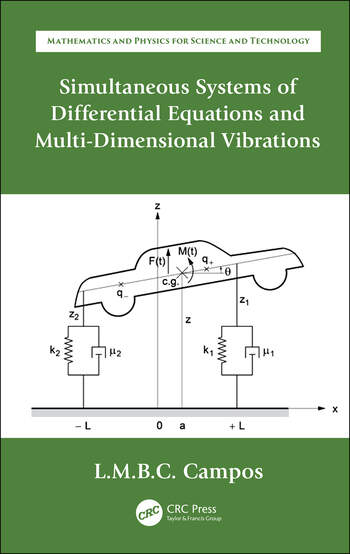# Simultaneous Systems of Differential Equations and Multi-Dimensional Vibrations

## 1st Edition

Luis Manuel Braga da Costa Campos

CRC Press
November 20, 2019 Forthcoming
Reference - 299 Pages - 34 B/W Illustrations
ISBN 9780367137212 - CAT# K409907
Series: Mathematics and Physics for Science and Technology

For Instructors Request Inspection Copy

was \$89.95

USD\$71.96

SAVE ~\$17.99

Availabe for Pre-Order. This item will ship after November 20, 2019
FREE Standard Shipping!

## Preview

### Summary

Simultaneous Differential Equations and Multi-Dimensional Vibrations is the fourth book within Ordinary Differential Equations with Applications to Trajectories and Vibrations, Six-volume Set. As a set, they are the fourth volume in the series Mathematics and Physics Applied to Science and Technology. This fourth book consists of two chapters (chapters 7 and 8 of the set).

The first chapter concerns simultaneous systems of ordinary differential equations and focuses mostly on the cases that have a matrix of characteristic polynomials, namely linear systems with constant or homogeneous power coefficients. The method of the matrix of characteristic polynomials also applies to simultaneous systems of linear finite difference equations with constant coefficients.

The second chapter considers linear multi-dimensional oscillators with any number of degrees of freedom including damping, forcing, and multiple resonance. The discrete oscillators may be extended from a finite number of degrees-of-freedom to infinite chains. The continuous oscillators correspond to waves in homogeneous or inhomogeneous media, including elastic, acoustic, electromagnetic, and water surface waves. The combination of propagation and dissipation leads to the equations of mathematical physics.

• Presents simultaneous systems of ordinary differential equations and their elimination for a single ordinary differential equation
• Includes cases with a matrix of characteristic polynomials, including simultaneous systems of linear differential and finite difference equations with constant coefficients
• Covers multi-dimensional oscillators with damping and forcing, including modal decomposition, natural frequencies and coordinates, and multiple resonance
• Discusses waves in inhomogeneous media, such as elastic, electromagnetic, acoustic, and water waves
• Includes solutions of partial differential equations of mathematical physics by separation of variables leading to ordinary differential equations

#### Instructors

We provide complimentary e-inspection copies of primary textbooks to instructors considering our books for course adoption.